Physics

Rope Swing Theory

Viscoelastic Theory behind Rope Swings
with numerical solutions of the Euler Lagrange Equations and real jumps from a bridge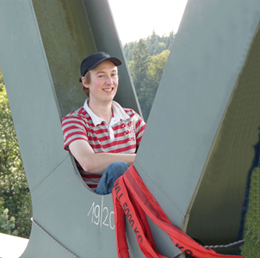Abstract: On this page I want to discuss the theory that lies behind rope swings. On Youtube one is able to discover lot´s of rope swing videos. The most famous video is "World's largest Rope Swing", filmed by Devin Graham: http://www.youtube.com/watch?v=4B36Lr0Unp4 It´s really an incredible feeling rushing downwards without feeling any force for about 35m. However what about the g-forces you have to withstand during such a swing? Is the rope damaged after a few jumps and what maximum forces are we deeling with? Is it better to use static ropes instead of standard climbing ropes? These and further questions I want to answer here... Max Bigelmayr, Sept. 2012, March 2013

1. Viscoelastic Model of a Rope Swing

1.1 Lagrange Equation and Dissipation Function

The pendulum is easily calculated with the help of Polar Coordinates. Let´s start with by discribing the mass-point, dependent on the angle P and the elongation y2. The derivation of the mass-point in realtion to time, gives us the speed vector of the mass-point as well as its absolut value squared: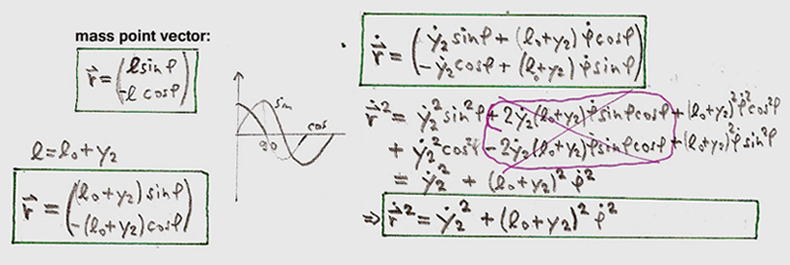The potential energy is seperated into Spring Energy and Hight Energy. The elongation of spring 2 with the factor D2, equals the realtiv movement y2-y1: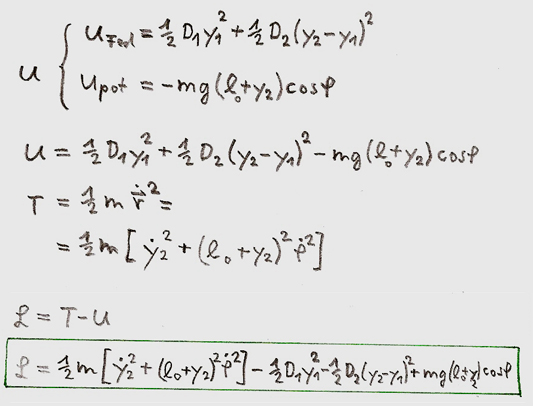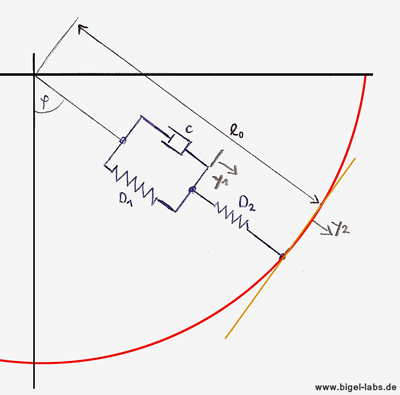Viscoelastic Pendulum with elongations y1 and y2
 1.2 Euler Lagrange Equations (Dissipation Function) Relaigh suggested a modification of the standard Euler Lagrange Equation which included a Dissipation Function D: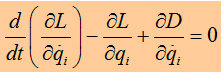The Dissipation Function D is defined by Stokes-Friction as follows: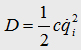, While the dissipation energy is discribed as: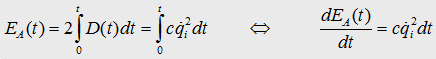. c is a constant which discribes the viscosity of the entire rope. Its unit is defined as [N/ms]. Now we can formulate the three differential equations for y1, y2 and P. Let´s start with the inner expansion y1: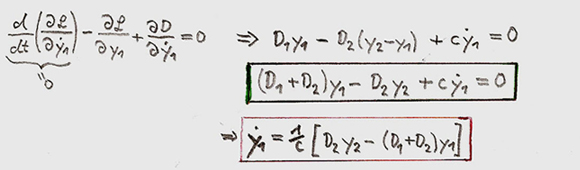equ.(1) The boundery condition for the given equation is: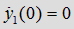and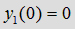______________________________________________________________________________________________ The differential equation for the outer expansion y2 is: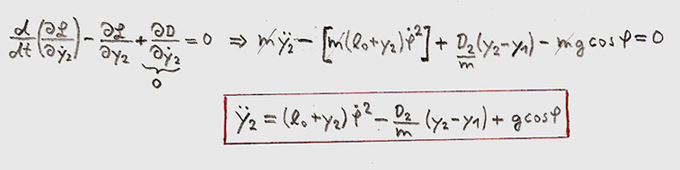equ. (2) The boundery condition in regards to this equation has to be found concerning the freefall, which occurs prior to the experience of rope force exerted on the person jumping. Thus the velocity vector of the freefall has to be smooth at this critical point. If you define the precise time at which the mass-point reaches its critical point, tcritical point=tcp=0, the boundery condition of y2(t) becomes apparent:But you also have to bare in mind the velocity vector. Remember the mass-point-movement-vector: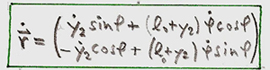At this critical point the following boundary condition is given, where the speed must be smooth: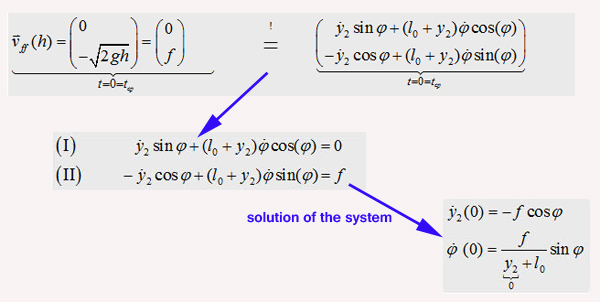So we are provided with the boundary condition for the start speed and start angle: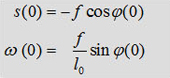_____________________________________________________________________________________________________ The derivation of Phi is as follows: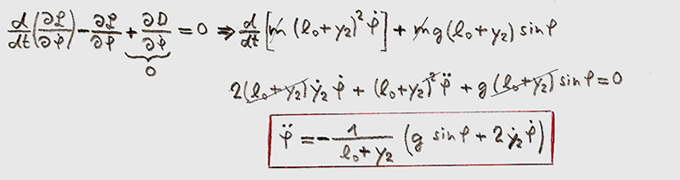equ. (3) ____________________________________________________________________________________________________ The acceleration a(t) of the rope equals d^2y/dt^2 [equ.(2)]. The function da(t)/dt is discribed by the following: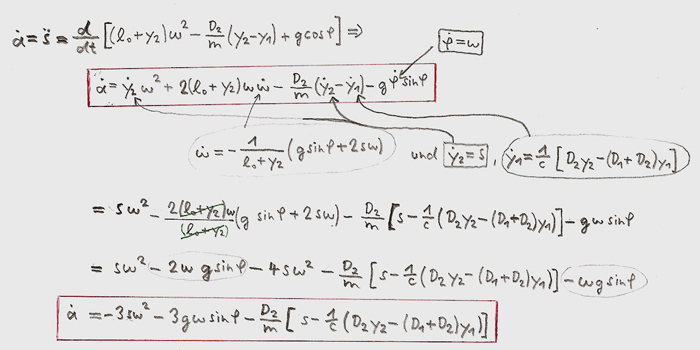The boundary condition for da/dt is: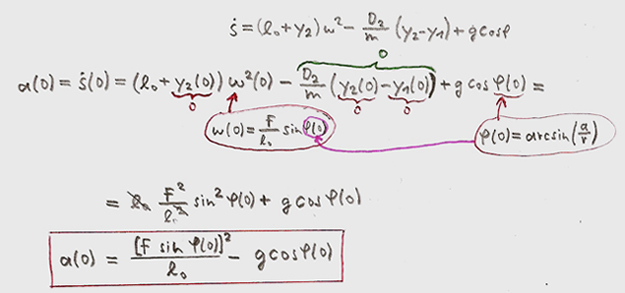1.3 Table of equations In the following table all the required equations and boundary conditions are summarized.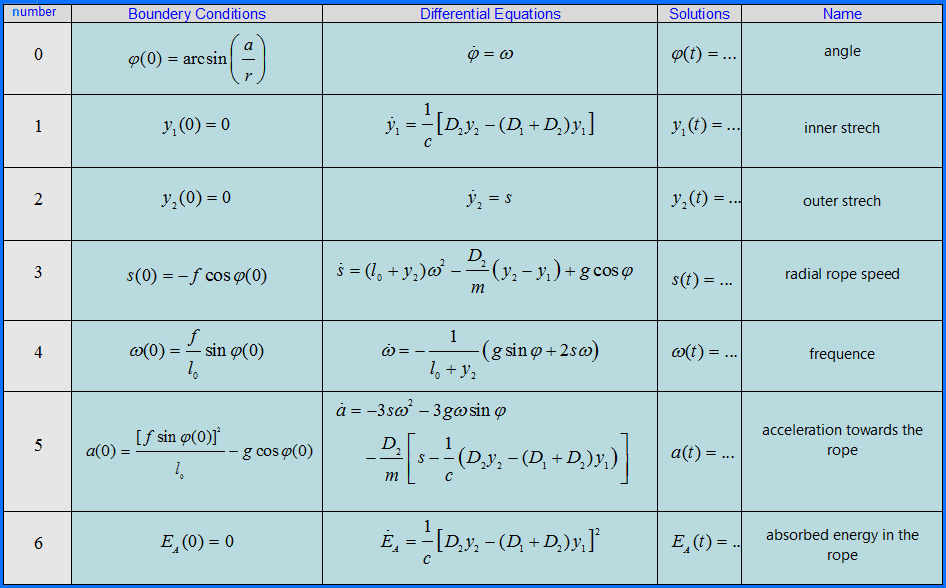2. Numerical Simulations
2.1 Elasticity moduls and typical rope-climbing parameters

 So now let´s look at some numerical simulations. For calculations it´s usefull to work with lenght independent constants. Therefore let`s use the elasticity modul E1 and E2 in place of D1 and D2: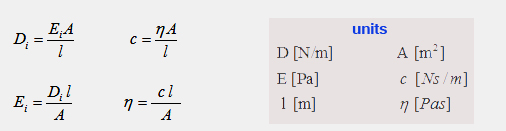In respect to the Viscoelastic Theory of Climbing Ropes [Ulrich Leuthäusser] one is able to discover the typical parameters of a climbing rope. These are: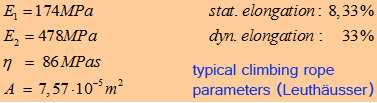Within the Graphs on the right you are able to view the oscillations of a climber [m=80kg] falling into the rope from falling height of 5m. The curves were numerically calculated with a Cash Carp 5th order system.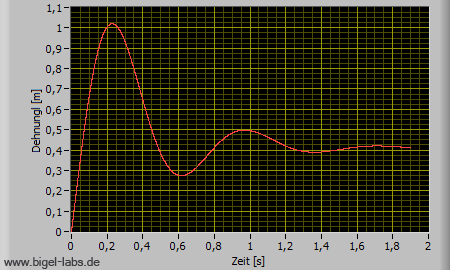Select one of the tabs bellow in order to view the graph you`re interested in: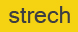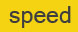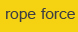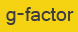But now let´s come back to the physics behind the rope-swing. If you´re lucky [or bad luck??? ;-)] you know a very large bridge like this: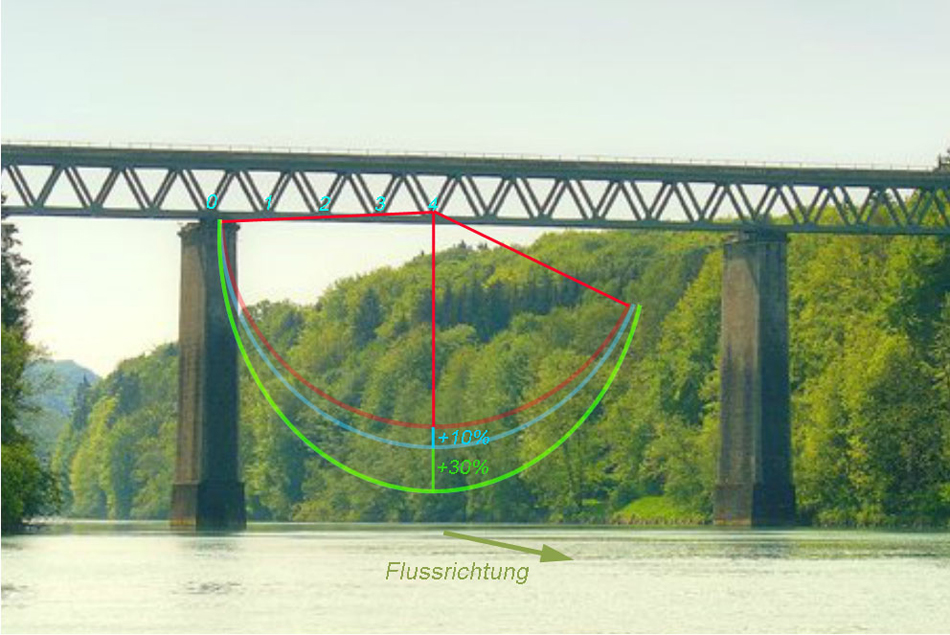Some people enjoy falling a long way down before experiencing the ropes tension. This brings up the interesting question as to the maximum g-force that a jumper has to withstand during this stage of the rope-swing.

.2.2 Programm and numerical simulations

I programmed a tool with that it´s possible to analyse the fall paths in detail. All the seven differential equations may be solved by the programm numerically by a Cash Carp Algorithm. At first you have to put in the precise, simulation time, intial time step. After defining the rope parameters [lenght, cross section, elaticity modul E1, E2, constant n (Pas)] and the jumper mass you may start the simulation.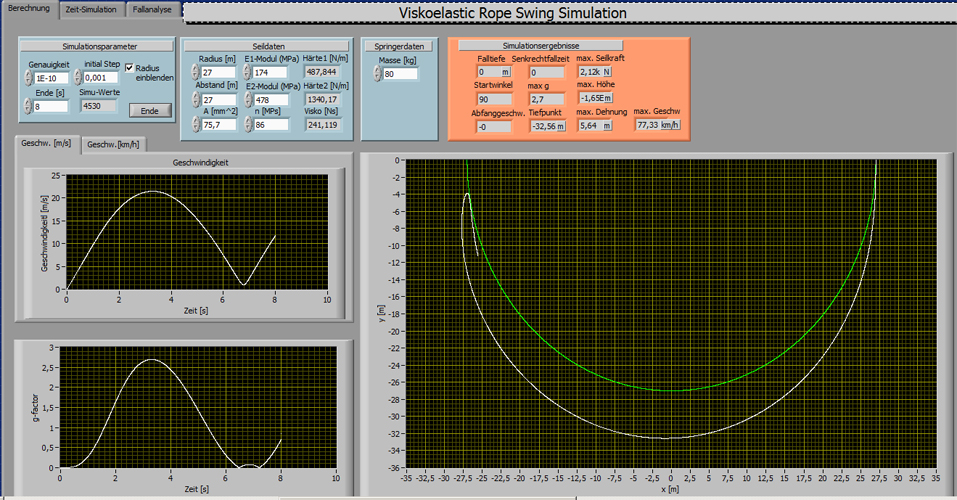Screenshot of the Rope Swing Simulation programm.

In the next step you may wath the falling paths and all the forces, speeds etc. in realtime. Annother option is to analyse specific points of the fall path in detail. [f.e. ]

2.3 Fall Path simulations

The following Simulations show the paths of a 75kg person jumping with a 27m long standart climbing rope [E1=174MPa, E2=478MPa, n=86MPas, A=7,57*10^-5m^2] at different jump distances:

Jump Distance 27m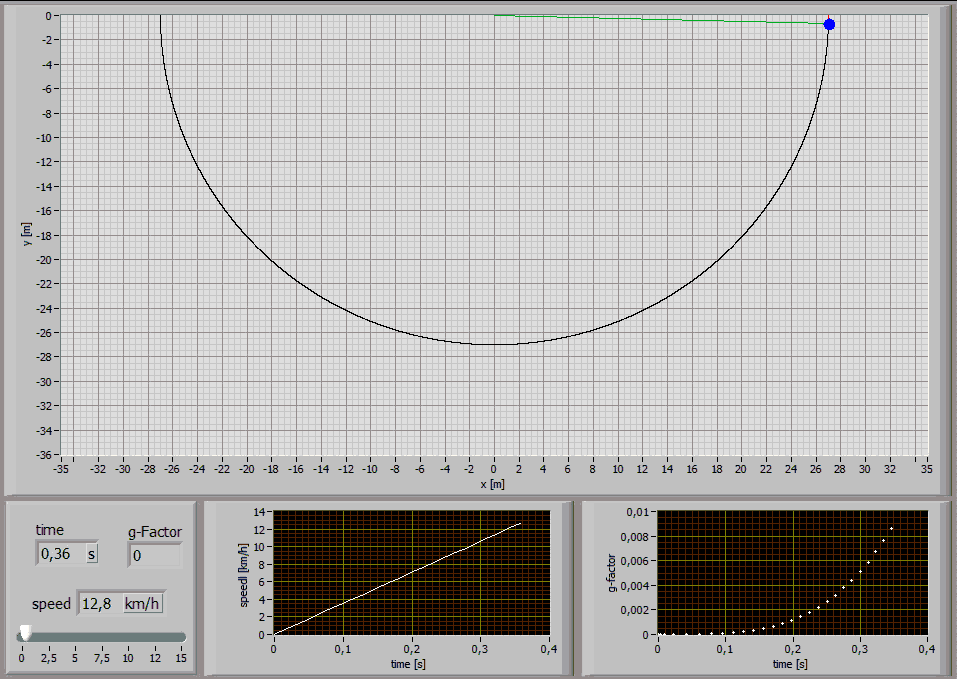Jump Distance 24m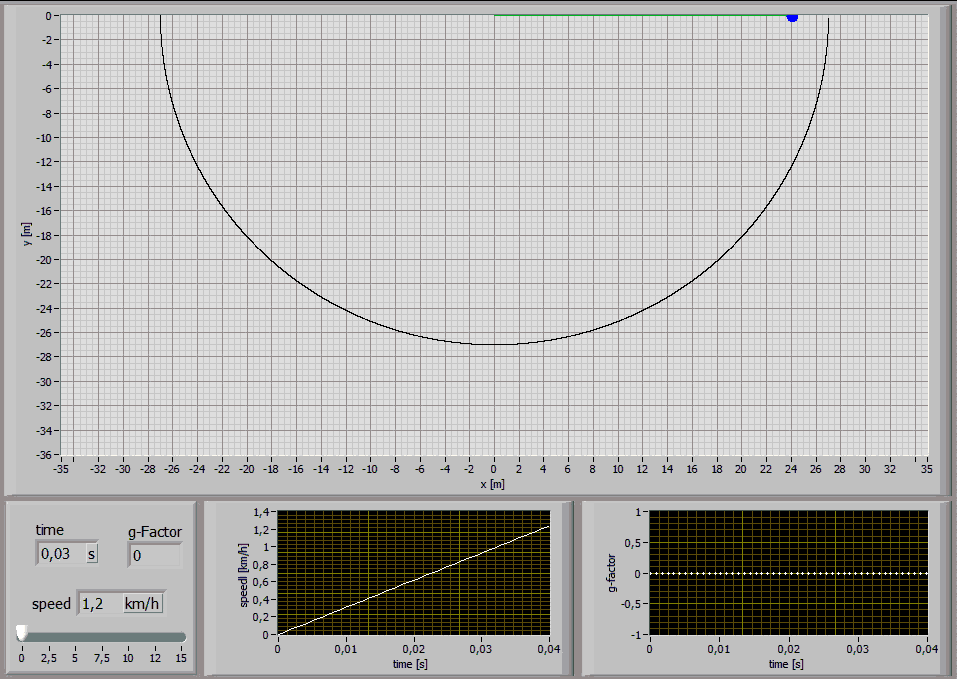Jump Distance 20m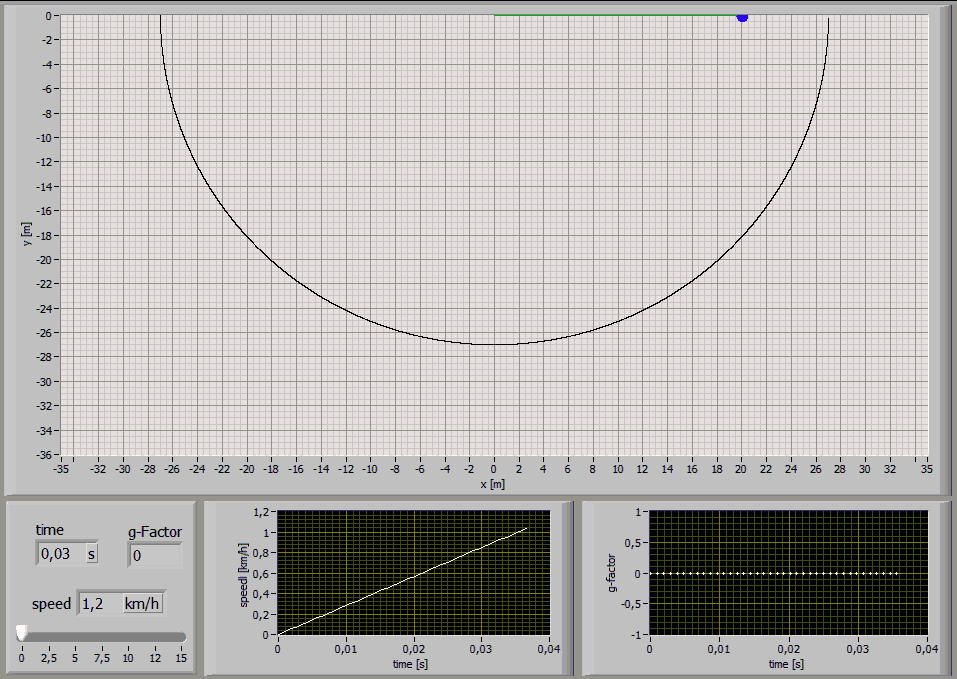Jump Distance 15m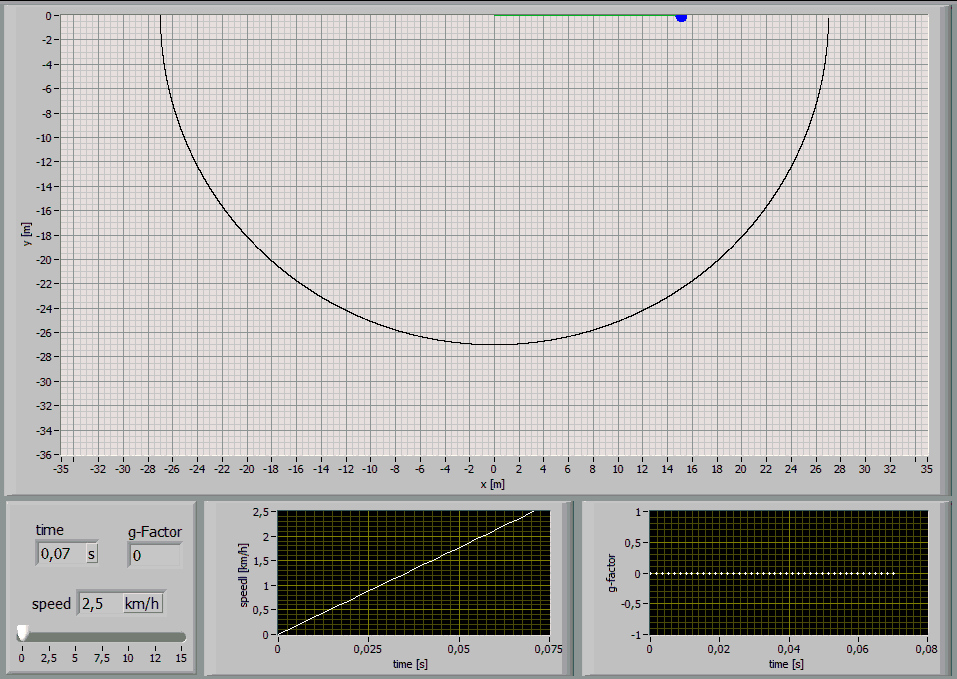Jump Distance 10m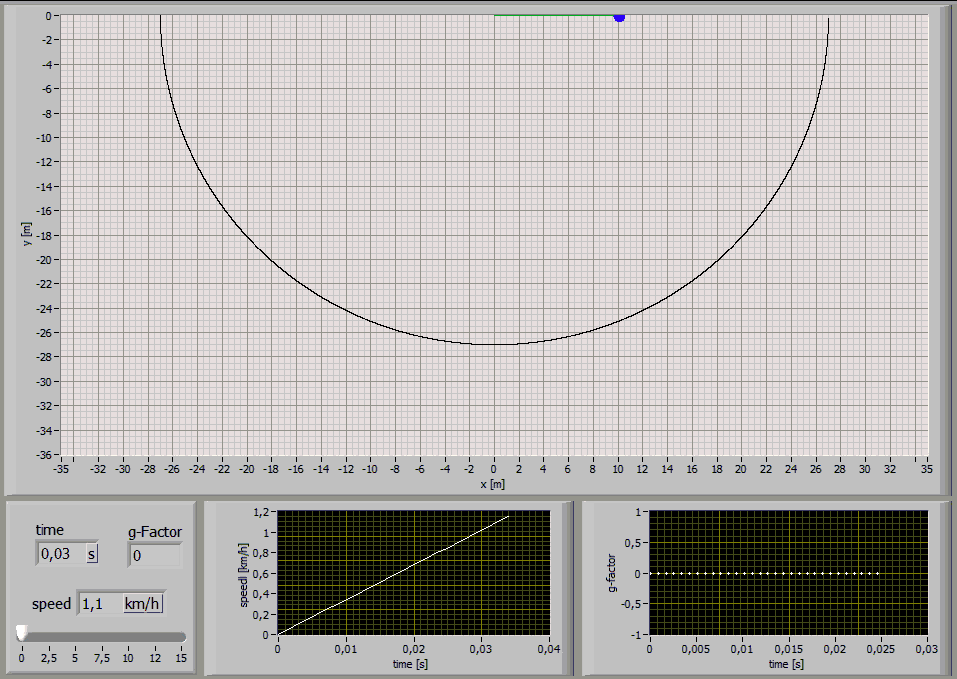Jump Distance 5m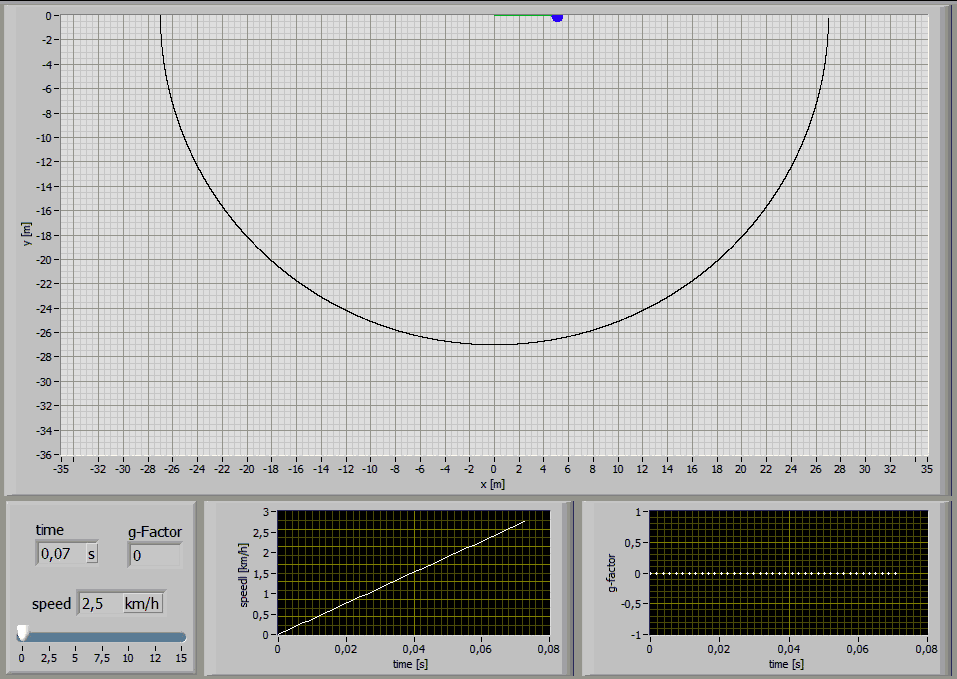2.4 Analysis of characteristic points

While a rope swing the g-force maxima are interesting. These graphs show the values for a 75kg person jumping with a 27m long standart climbing rope [E1=174MPa, E2=478MPa, n=86MPas, A=7,57*10^-5m^2] at a jump distance of 24m:2.5 g-Factor calculations

By analysing the maximum g-forces at certain jump distances one gets the following distributions: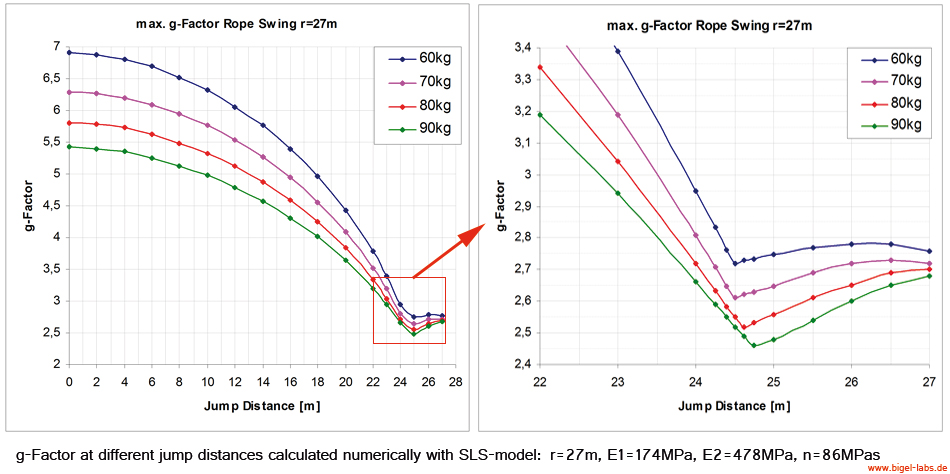It´s quite interesting that there is a certain jump distance beween 24m and 25m where the jumping person has to withstand minimum g-forces during the swing.
So it´s better for dorsal to jump at around 24m.

3. Rope live-time approximation

Martyn Pavier [University of Bristol, Department of Mechanical Engineering] did some Experimental and theoretical simulation of climbing falls. He found a logaritmic dependence of the maximum tension and the number of falls to failure [graph on the right hand sight]: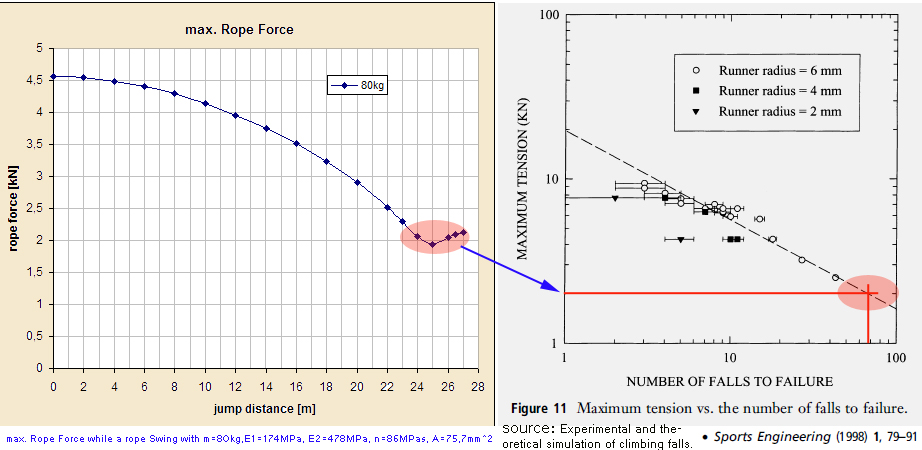Graph1: Numerical claculation of the maximum rope force while a rope swing at different jump distances Graph2: Maximum tension vs. the number of falls to failure measured by Martyn Pavier

Let´s assume a rope swing jumper which has got a jump distance of around 23-27m while his jumps[rope lenght 27m]. As Graph1 shows the maximum rope force while the swinging is around 2kN. Comparison of this value with the number of fails M. Pavier found one may assume that a standart climbing rope will fail after 50-80 jumps.
Depending on the "history" of the rope the number of jumps to failure may also be even less than 50 jumps. So it is allways a must to use a redundance rope!

4. Real jumps

After the simulations it´s time for real jumps. We had the luck to found a 36m hight bridge...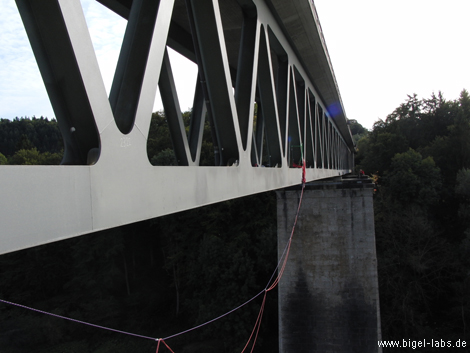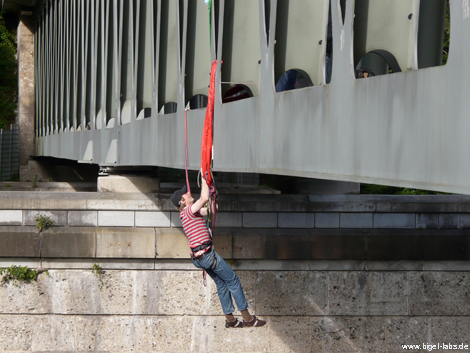Three rope system. Preparing of the "Anker point" 36m above the ground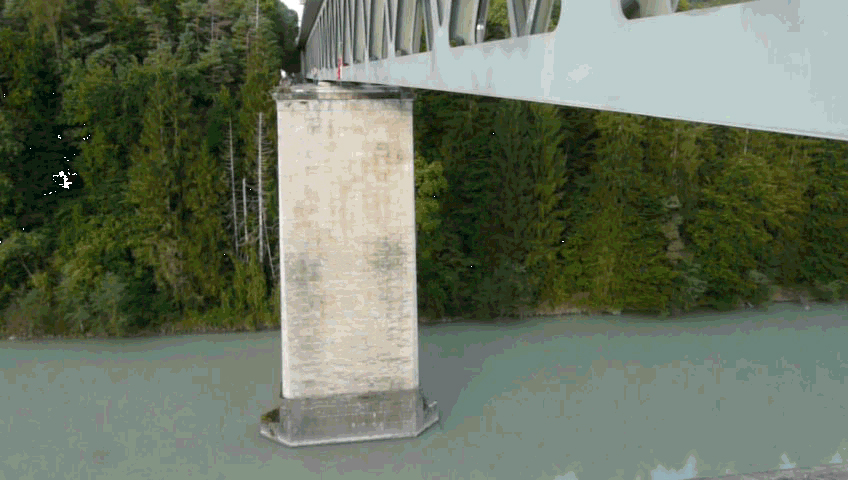First jump of my friend Markus [click here to watch the gif.]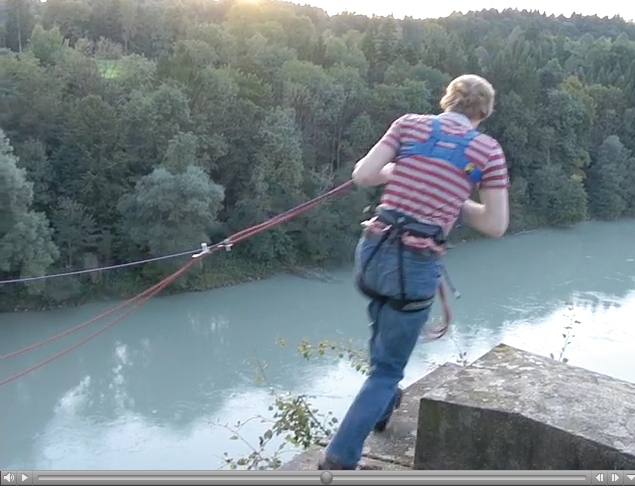AVI-Film of my first jump [you may watch with "click".. ]

5. Discussion

The calculations and simulations which are presented here are a usefull to get a first approximation of the forces a rope swing jumper has to withstand during the swing.
Air resistance and rope mass are not respected in the simulations yet. Next steps will include:
- Finding the differential equations including air resistance effects
- Multi Rope Analysis
- Static Rope Analysis
- Rope Failure Simulations [redundance rope behavior after a failure of the main rope]
- Experimental studies and fall experiments with steel mass falling into climbing ropes to get the exact rope parameters

So there is a lot to do....;-)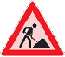Last update:
March 2013